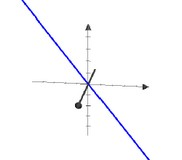# Geometry - Shape (with transformation and notion)

Viz - Graphic Object in Geometry

A shape is any list of line drawing commands, which strung together, describe a continuous line or closed shape that can be filled or stroked.

• Move,
• Line,

## Predefined

Predefined shapes (ie not arbitrary shapes) that can be constructed with their properties (ie it's not needed to use a path operator to define them)

The following geometric object (point, line, plane) contains the origin before translation.

Discover MoreGeometry - Circle

A geometric object. There is 360 degrees in a circleGeometry - Ellipse

An ellipse is a predefined shape. Under shear, a circle becomes an ellipse.Geometry - Object

in Geometry An object can be: a pre-defined shape or an arbitrary one defined with the help of a path. Notions such as: The length of a vector The angle between two vectors. are introduced...Geometry - Origin

All geometrics objects definition goes through the origin. In order to go through an another point, a translation (addition) must be performed.Geometry - Parallelogram

A geometric object.Image Vector - Path

A path is a graphic object Paths define: an arbitrary shapes, line trajectories, and regions A path may intersect itself and may have disconnected sections and holes. Programs use paths to draw...Linear Algebra - Find intersection of geometric objects

Find intersection of geometric objects Find the intersection of the plane spanned by [1, 0, 0] and [0, 1,−1] the plane spanned by [1, 2,−2] and [0, 1, 1] The orthogonal complement in of...Linear Algebra - Inner product of two vectors

Inner products allow the rigorous introduction of intuitive geometrical notions such as the length of a vector or the angle between two vectors. They also provide the means of defining orthogonality between...Linear Algebra - Vector Space (set of vector)

A vector space is a subset of the set of function representing a geometric objectpassing through the origin. A vector space over a field F is any set V of vector : with the addition and scalar-multiplication...SVG - Shape and Path

The representation of geometric object (shape) in svg. They are in the grammar. You need to use the path element to create them